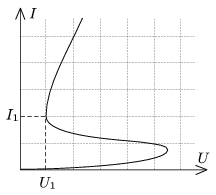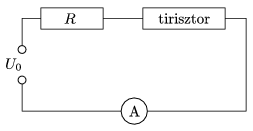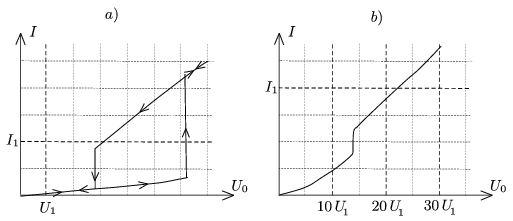Mathematical and Physical Journal
for High Schools
Issued by the MATFUND Foundation
 Already signed up? New to KöMaL?

#Problem P. 4549. (May 2013)

P. 4549. A thyristor is an electrical element made of semiconductor, and can be used in many different ways. Its current-voltage characteristics is quite strange. (See figure 1.)The thyristor is connected as shown in figure 2, and the voltage U0 is slowly increased from zero to Umax , and then it is slowly decreased back to zero.Graph the current as a function of the voltage U0, if

a)and,

b)and.

(5 pont)

Deadline expired on June 10, 2013.

Sorry, the solution is available only in Hungarian. Google translation

Megoldásvázlat.A tirisztor $\displaystyle U(I)$ nemlineáris karakterisztikájának és az $\displaystyle U(I)=U_0-IR$ egyenesnek (ún. munkaegyenesnek) a metszéspontja adja meg a keresett $\displaystyle I(U_0)$ kapcsolatot. (Több metszéspont esetén a meredekségek nagysága választja ki a stabil állapotot.)

### Statistics:

 12 students sent a solution. 5 points: Asztalos Bogdán, Olosz Balázs, Papp Roland. 4 points: Beregi Ábel, Szilágyi András. 3 points: 3 students. 2 points: 2 students. 0 point: 2 students.

Problems in Physics of KöMaL, May 2013